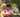### Odd Occurrences In Array

January 16, 2020

Odd Occurrences In Array

Write a function:
function solution(A);
that, given an array A consisting of N integers, returns the value of the unpaired element.

For example, given array A such that:
A = 9
A = 3
A = 9
A = 3
A = 9
A = 7
A = 9
the function should return 7.
Write an efficient algorithm for the following assumptions:
• N is an odd integer within the range [1..1,000,000];
• each element of array A is an integer within the range [1..1,000,000,000];
all but one of the values in A occur an even number of times.

``````function solution(A) {
// write your code in JavaScript (Node.js 8.9.4)
A.sort()
for(let i=0; i<A.length; i+=2){
if(A[i]!=A[i+1]) return A[i]
}
return A[A.length-1]
}
console.log("solution([9,3,9,3,9,7,9]): ", solution([9,3,9,3,9,7,9]))``````Posted by Tai Lu ( 呂台生 ) who's building useful things.You should follow him on Twitter